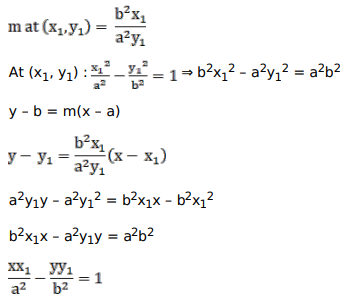# Show that the equation of the tangent to the hyperbola

Question:

Show that the equation of the tangent to the hyperbola $\frac{x^{2}}{a^{2}}-\frac{y^{2}}{b^{2}}=1$ at $\left(x_{1}, y_{1}\right)$ is $\frac{x_{1}}{a^{2}}-\frac{y y_{1}}{b^{2}}=1$.

Solution: